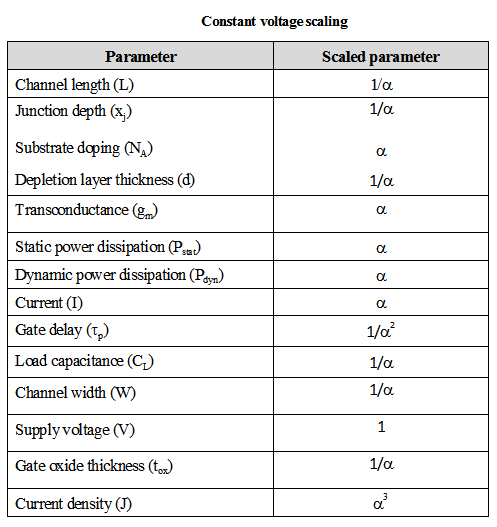Home > Digital CMOS Design > CMOS Layout Design > Types of Scaling

Types-of-Scaling

Types of Scaling :

There are three types of scaling as constant voltage, constant field and lateral scaling. In constant voltage scaling, VDD is kept constant, and the process is scaled. For constant field scaling, the device dimensions are scaled by the parameter λ.

Constant Field Scaling :

In constant field scaling the scaled devices are obtained by scaling all dimensions of transistor, device voltages and the doping concentration densities by factor . The effect of scaling is shown in Table belowTypes-of-Scaling

From the table it is seen that the device dimension, L, W, tox, xj, NA are scaled by factor . The most important point in this scaling is the supply voltage is scaled but the electric field remains constant hence the same constant field scaling is given.

Constant Voltage Scaling :

• In constant voltage scaling the supply voltage VDD is kept constant while the process is scaled. The effect of scaling is shown in Table below.Types-of-Scaling

• With constant voltage scaling the electric field increases which has lead to the development of the lateral double diffused structures.

Lateral Scaling : In lateral scaling only the gate length is scaled. This is also called as the "gate shrinking". The effect of this scaling of parameters is shown in Table below.Types-of-Scaling